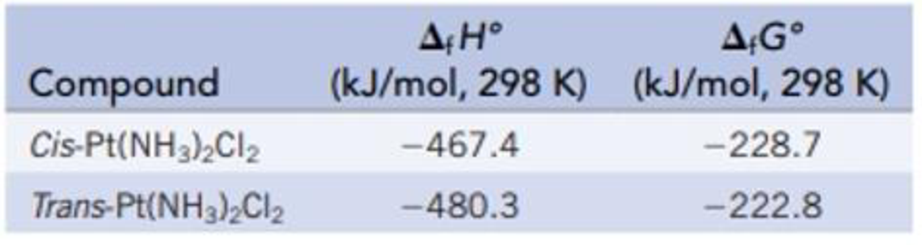Chapter 18, Problem 84IL

Chapter
Section
Textbook Problem

Cisplatin [cis-diamminedichloroplatinum(II)] is a potent treatment for certain types of cancers, but the trans isomer is not effective. What is the equilibrium constant at 298 K for the transformation of the cis to the trans isomer? Which is the favored isomer at 298 K, the cis or the trans isomer?Interpretation Introduction

Interpretation:

The equilibrium constant for the transformation of cisplatin to transplatin at 298 K should be calculated.

Concept introduction:

The Gibbs free energy or the free energy change is a thermodynamic quantity represented by ΔrGo. It can be calculated in a similar manner as entropy and enthalpy. The expression for the free energy change is:

ΔrG°fG°(products)fG°(reactants)

It is related to entropy and entropy by the following expression,

ΔrGorHo-TΔrSo

Here, ΔrH° is the change in enthalpy and ΔrS° is the change in entropy.

ΔrGo is related to the equilibrium constant Kp by the equation,

ΔrGo= -RTlnKp

The rearranged expression is,

Kp= eΔrGoRT

Explanation

The equilibrium constant for the transformation of cisplatin to transplatin at 298 K is calculated below.

Given:

The given reaction is,

CisplatinTransplatin

The ΔrGo for cisplatin is 228.7 kJ/mol.

The ΔrGo for transplatin is 222.8 kJ/mol.

ΔrG°fG°(products)fG°(reactants)=[(1 mol Transplatin/mol-rxn)ΔfG°[

Still sussing out bartleby?

Check out a sample textbook solution.

See a sample solution

The Solution to Your Study Problems

Bartleby provides explanations to thousands of textbook problems written by our experts, many with advanced degrees!

Get Started